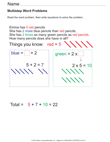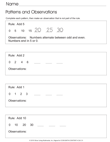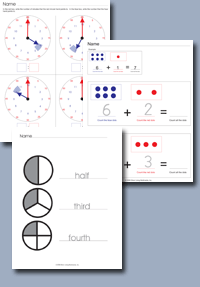﻿ Common Core Standards - Operations and Algebraic Thinking Grade 4Adaptive Worksheets

Helping Every Child to Succeed

toll-free (888) 777-0876
fax (888) 777-0875

## Operations and Algebraic Thinking for Grade 4

### Use the four operations with whole numbers to solve problems.

• CCSS.MATH.CONTENT.4.OA.A.1
Interpret a multiplication equation as a comparison, e.g., interpret 35 = 5 × 7 as a statement that 35 is 5 times as many as 7 and 7 times as many as 5. Represent verbal statements of multiplicative comparisons as multiplication equations.

• CCSS.MATH.CONTENT.4.OA.A.2
Multiply or divide to solve word problems involving multiplicative comparison, e.g., by using drawings and equations with a symbol for the unknown number to represent the problem, distinguishing multiplicative comparison from additive comparison.

• CCSS.MATH.CONTENT.4.OA.A.3
Solve multistep word problems posed with whole numbers and having whole-number answers using the four operations, including problems in which remainders must be interpreted. Represent these problems using equations with a letter standing for the unknown quantity. Assess the reasonableness of answers using mental computation and estimation strategies including rounding.

•Multistep Word Problems

### Gain Familiarity with Factors and Multiples

• CCSS.MATH.CONTENT.4.OA.B.4

Find all factor pairs for a whole number in the range 1-100. Recognize that a whole number is a multiple of each of its factors. Determine whether a given whole number in the range 1-100 is a multiple of a given one-digit number. Determine whether a given whole number in the range 1-100 is prime or composite.

### Generate and Analyze Patterns

• CCSS.MATH.CONTENT.4.OA.C.5

Generate a number or shape pattern that follows a given rule. Identify apparent features of the pattern that were not explicit in the rule itself.Patterns and Observations